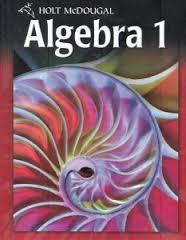# Algebra 1 Lap 3 Quiz 2 Practice

28 Questions | Attempts: 751
ShareSettings.

• 1.
Describe the correlation in the given relationship.The distance a person runs and how physically tired that person is.
• A.

Positive correlation

• B.

Negative correlation

• C.

No correlation

• 2.
Describe the correlation in the given relationship.The number of cats in a barn and the number of mice in that barn.
• A.

Positive correlation

• B.

Negative correlation

• C.

No correlation

• 3.
Describe the correlation in the given relationship.The number of miles driven and the number of gallons of gas left in the tank.
• A.

Positive correlation

• B.

Negative correlation

• C.

No correlation

• 4.
Describe the correlation in the given relationship.The age of a person and the amount of broccoli that person eats.
• A.

Positive correlation

• B.

Negative correlation

• C.

No correlation

• 5.
Describe the correlation in the given relationship.The number of first downs an offense makes in a football game and the number of punts in that game.
• A.

Positive correlation

• B.

Negative correlation

• C.

No correlation

• 6.
Which of the following graphs shows a positive correlation?
• A.

Graph A

• B.

Graph B

• C.

Graph C

• D.

Graph D

• 7.
Which of the following graphs shows a negative correlation?
• A.

Graph A

• B.

Graph B

• C.

Graph C

• D.

Graph D

• 8.
Which of the following graphs might best show the length of a pencil as a function of the number of times it is sharpened?
• A.

Graph A

• B.

Graph B

• C.

Graph C

• D.

Graph D

• 9.
Which of the following graphs might best show the grade average of a student as a function of the number of hours spent studying?
• A.

Graph A

• B.

Graph B

• C.

Graph C

• D.

Graph D

• 10.
Which of the following graphs might best show the frequency of flossing teeth and number of trips to eye doctor?
• A.

Graph A

• B.

Graph B

• C.

Graph C

• D.

Graph D

• 11.
Determine whether the sequence appears to be an arithmetic sequence.  If so, find the common difference.−1, −3, −9, −27, ...
• A.

Not an arithmetic sequence

• B.

Arithmetic sequence; common difference is 3

• C.

Arithmetic sequence; common difference is −3

• 12.
Choose the scatter plot that best represents the relationship between the number of movie tickets sold and the amount of concession sales.
• A.

Graph A

• B.

Graph B

• C.

Graph C

• D.

Graph D

• 13.
Choose the scatter plot that best represents the relationship between the number of movie tickets sold and the number of available seats.
• A.

Graph A

• B.

Graph B

• C.

Graph C

• D.

Graph D

• 14.
Determine whether the sequence appears to be an arithmetic sequence.  If so, find the common difference.3, −2, −7, −12, …
• A.

Not an arithmetic sequence

• B.

Arithmetic sequence; common difference is 3

• C.

Arithmetic sequence; common difference is −5

• 15.
Determine whether the sequence appears to be an arithmetic sequence.  If so, find the common difference.2, 4, 8, 16…
• A.

Not an arithmetic sequence

• B.

Arithmetic sequence; common difference is 2

• C.

Arithmetic sequence; common difference is 16

• 16.
Determine whether the sequence appears to be an arithmetic sequence.  If so, find the common difference.165, 150, 135, 120, …
• A.

Not an arithmetic sequence

• B.

Arithmetic sequence; common difference is 15

• C.

Arithmetic sequence; common difference is −15

• 17.
​Find the 60th term of the arithmetic sequence.−20, −8, 4, 16, …
• 18.
​Find the 35th term of the arithmetic sequence.32, 35, 38, 41, …
• 19.
Find the 41st term of the arithmetic sequence given a1 = 120 and d = −7.
• 20.
Find the 21st term of the arithmetic sequence given a1 = 24 and d = −3.
• 21.
​Find the 19th term of the arithmetic sequence given a1 = −200 and d = −7.
• 22.
​Find the 44th term of the arithmetic sequence.120, 110, 100, 90, …
• 23.
​Find the 40th term of the arithmetic sequence.−40, −38, −36, −34, …
• 24.
​Find the 67th term of the arithmetic sequence.−32, −24, −16, −8, …
• 25.
Mark’s new job has a starting salary of \$39,000 and annual increase of \$1200.  How much will he earn during his 15th year?
• A.

\$55,800

• B.

\$57,000

• C.

\$58,200

• D.

\$59,400

## Related TopicsBack to top
×

Wait!
Here's an interesting quiz for you.